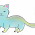### What's Wrong? Q9c

1.-3 * -3 is -9 not 6

2.-3 X -3 is -9 not 6

3.Should be 9 not 6...

4.-3 * -3 = 9 not 6

5.Instead of multiplying the 3 by itself, the person added instead.

6.calculation error: -3 x -3 = 9

7.-3y*-3y=9y^2

8.Again, this is me (Haiyo, so embarrassing*auntie lucy action*)
Err... Careless. 3X3 shld be 9 instead of 6. Careless agn. >.<

9.-3x-3=9 not 6

10.calculation mistake, -3 x -3=9, not 6

11.Careless?
-3y x -3y= 9y^2 (not 6y^2)

12.3 x -3 is -9 not 6

13.3X3 equals 9 not 6 the person may have took it as a plus

14.The student calculated wrongly. -3y X -3y is equal to 9y^2 not 6y^2

15.-3y X -3y = 9y^2

16.This was a calculation error

17.There was a calculation mistake in the equation, (-3y)(-3y) is 9y^2 and not 6y^2 and why was there an underline for the expressions in the (6xy^2-9y^2)? Did not simplify?

18.-3 * -3 is 9 and not 6. (and not -9)

19.-3y x -3y is equal to 9y^2

20.(-3y)(-3y)= 9y^2

21.The student has done his/her calculating wrongly :(

22.(-3)(-3) = 9.

23.The student probably never include a bracket around -3 in the calculator.